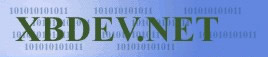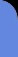Monday August 19, 2019
 home | about | contact | Donations

 SOS Data Encoding. by bkenwright@xbdev.nett   Well before you get to the SOS Section, you should have read in the data from SOF (Start of Frame) Section, which tells you how many Y block, U blocks and V blocks there are.   So using this information we start to read in our data.     /* Skip the bock of data at the start of the SOS which tells us how many componets there and which tables each component uses */   <- Start of our encode data begins here imagine-> Read in a Huffman code which can be of variable length bits… this is determined by going bit by bit through our data from the start until we find a matching code that is the same as in our Huffman table (e.g. Table ID: 0, Component: 0).   This Huffman code is compared with its equivalent value.. which turns out to be a single byte value. The byte tells us how many bits to read next… e.g. 6, so the next 6 bits is our image data.  We get the next six bits, and convert it to an unsigned char… can’t go having loads of bits of different lengths in our code.  As you should well know, 01 is the same as 0000 0001 in binary.   Well after the dc part comes all the ac components.  We read the data in ‘similarly’…but not the same.  First we read the Huffman code value, which can be of any number of bits… be keep reading the bits until we find a matching value in our Huffman table.  We then get the matching value with which it is equal… it should be a single byte. Now the upper nibble (top 4 bits) represents how many zero’s before our value, and the lower nibble (lower 4 bits) tells us how many bits to read in next to get our image value.   For example if the byte value we decode was 0x38, it would mean that the next three values are 0, and to read in the next 8 bits to get the image value that follows those three 0’s in our 64 array element.     Recap! I know a recap already your saying, well this is very important… I’m going to do some hex dumps later and show you the actual one’s and zero’s so you can get a feel of what is actually happening in jpeg file.       <- Starting at the beginning of our image data ->     Things to look for in the stream of data… if you get a   0xff value followed by 0x00, ignore the 0x00.   If the huf code decodes to 0x00, it means that all the rest of the 64 array are zero’s.           Binary Number Basics.   Standard binary counting… Number (10):               Binary Number(2): 1                                  01 2                                  10 3                                  11   Code length examples.   Coded Binary Values (1 bit) Number(10);                Binary Number(2): -1                                 0 (e.g. represented in 1 byte this would be 1111 1110 ). 1                                  1   Coded Binary Values (2 bits) Number(10):                Binary Number(2): -3                                 00 -2                                 01 2                                  10  3                                  11   Coded Binary Values (3 bits) Number(10):                Binary Number(2): -7                                 000 -6                                 001 -5                                 010 -4                                 011 4                                  100   5                                  101  6                                  110 7                                  111       Things you notice J Negative values start with a “1”, also the negative values are the one’s compliment of its positive value, e.g. invert all the bits.   So how do we get these values?  Well if you’ve got a calculator that does binary, type 3 in and convert it to binary, you’ll find the calculator displays 11, which is the minimum number of bits that can represent the binary number 3, so it takes 2 bits. Alternatively if we wanted we could represent 3 as 0000000000000011, and it would still mean the same value.   So all the value that where reading using the number of bits is the minimum representation of the number using binary without all the necessary padding bits.     Putting the minimum bits back into a byte container is easy as well. If the first bit is not a 1, we can just say:   unsigned char byte_var = bits values (e.g. 011);   if the first bit is negative, then we have to put all one’s in upto our value, then put our data in and add one (effectively a 2’s complement).   unsigned char byte_var = -1; // so byte_var contains all 1’s.                char_byte = char_byte << num bits (e.g. 3 for example). // so byte_var contains all one’s except the last number that we shifted (e.g. the last 3 will be zero if we shifted 3 times).                char_byte = char_byte + bits. // our char_byte has all ones and the bits values on the end.                char_byte = char_byte + 1. // effectively all the bits are inverted until we reach a 1.   Example 0101 (4 bits in length)…. 0 at the start so its negative…   1111 1111 shift left 4 bits and we get 1111 0000. Add our data bits to this value gives us, 1111 0101, and finally add one to it: 1111 0110.   If you check that on your calculator… 1111 0110, you’ll get? 246.. why not a negative number I hear you say…. well  1001 is 9, add 1 to it… so put –10 in your calculator and convert it to binary and you get 111..1110110 which is our but with loads of padded 1’s.   If first bit is positive then:     Bits + (  (-1)<
 Visitor: 9534626  { 209.237.238.175 } Copyright (c) 2002-2017 xbdev.net - All rights reserved. Designated tutorial and software are the property of their respective owners.